# 18  Parametric Survival Models

## 18.1 Homogeneous Models (No Predictors)

Why use a parametric model?

1. easily compute selected quantiles of the survival distribution
2. estimate (usually by extrapolation) the expected failure time
3. derive a concise equation and smooth function for estimating $$S(t)$$, $$\Lambda(t)$$, and $$\lambda(t)$$
4. estimate $$S(t)$$ more precisely than $$S_{\rm KM}(t)$$ or $$S_{\Lambda}(t)$$ (Altschuler-Nelson-Fleming-Harrington estimator) if the parametric form is correctly specified.

Note: Fitting more than two smooth survival curves and choosing the one that best reproduces the KM estimator will result in a true precision no better than KM due to model uncertainty.

### 18.1.1 Specific Models

• Seen exponential and Weibull already
• Many others obtained by assuming $$\log(T)$$ has a certain dist.
• Log-normal: $$S(t) = 1 - \Phi(\frac{\log(t)-\mu}{\sigma})$$
• Log-logistic: $$S(t) = [1 + \exp(-\frac{\log(t)-\mu}{\sigma})]^{-1}$$
• Log-extreme value: $$S(t) = \exp[-\exp(\frac{\log(t)-\mu}{\sigma})]$$
another way of expressing Weibull

### 18.1.2 Estimation

• Log-likelihood for exponential distribution

$\log L = \sum_{i:Y_{i} {\rm\ uncensored}}^{n} \log \lambda - \sum_{i=1}^{n} \lambda Y_{i}$

$\begin{array}{ccc} \hat{\lambda} &=& n_{u}/w \\ {\rm var}(\hat{\lambda}) &=& n_{u}/w^{2} \\ {\rm var}(\log \hat{\lambda}) &=& 1/n_{u} \\ \hat{\mu} &=& w/n_{u} \\ \hat{S}(t) &=& \exp(-\hat{\lambda}t) \end{array}$

Consider these failure time data:

$\begin{array}{c} 1 \ \ 3\ \ 3\ \ 6^{+}\ \ 8^{+}\ \ 9\ \ 10^{+} . \nonumber \end{array}$
Code
require(rms)
S <- Surv(c(1, 3, 3, 6, 8, 9, 10), c(1,1,1,0,0,1,0))
fe <- psm(S ~ 1, dist='exponential')
f2 <- psm(S ~ 1, dist='weibull')
$\begin{array}{ccc} n_{u} &=& 4 \\ w &=& 40 \\ \hat{\mu} &=& 10 \pm 5 \\ T_{0.5} &=& 10 \log(2) \end{array}$
• Weibull fit
$\begin{array}{ccc} \hat{\alpha} &=& 0.0728 \nonumber \\ \hat{\gamma} &=& 1.164 \nonumber \\ \hat{S}(t) &=& \exp(-0.0728t^{1.164}) \\ \hat{S}^{-1}(0.5) &=& [(\log 2)/\hat{\alpha}]^{1/\hat{\gamma}} = 6.935 \\ {\rm (estimated\ median)} \nonumber \end{array}$

### 18.1.3 Assessment of Model Fit

• Example: Weibull

$\log[-\log S(t)] = \log \Lambda(t) = \log \alpha +\gamma (\log t)$

• Plot $$\log \hat{\Lambda}(t)$$ versus $$\log t$$
• For assumed dist. $$S(t)$$ plot $$S^{-1}[S_{\Lambda}(t)]$$ or $$S^{-1}[S_{\rm KM}(t)]$$ against $$t$$, check for linearity
• Log-distributions: plot vs. $$\log t$$
• Check log-normal: plot $$\Phi^{-1}[S_{\Lambda}(t)]$$ vs. $$\log t$$
• Check log-logistic: plot $${\rm logit}[S_{\Lambda}(t)]$$ vs. $$\log t$$
• Alternative: plot fitted $$\hat{S}(t)$$ and $$S_{\Lambda}(t)$$ vs. $$t$$ on the same graph

## 18.2 Parametric Proportional Hazards Models

### 18.2.1 Model

$\lambda(t|X) = \lambda(t) \exp(X\beta)$

$\begin{array}{ccc} \Lambda(t|X) &=& \Lambda(t) \exp(X\beta) \nonumber \\ S(t|X) &=& \exp[-\Lambda(t)\exp(X\beta)] = \exp[-\Lambda(t)]^{\exp(X\beta)} \end{array}$

$S(t|X) = S(t)^{\exp(X\beta)} ,$

### 18.2.2 Model Assumptions and Interpretation of Parameters

$\begin{array}{ccc} \log \lambda(t|X) &=& \log \lambda(t)+X\beta \nonumber \\ \log \Lambda(t|X) &=& \log \Lambda(t)+X\beta \end{array}$

Assumptions:

• Underlying functions ($$\lambda$$, $$\Lambda$$, $$S$$)
• Linear effect of predictors on $$\log\lambda$$, $$\log\Lambda$$
• No interaction between $$X$$ and $$t$$ $$\rightarrow$$ impact same over time
$\begin{array}{ccc} \beta_{j} &=& \log \lambda(t|X_{1}, X_{2}, \ldots, X_{j}+1, X_{j+1}, \ldots, X_{k}) \\ &-& \log \lambda(t|X_{1}, \ldots, X_{j}, \ldots, X_{k}) , \end{array}$
• Effect of increasing $$X_{j}$$ by $$d$$ is to increase $$\lambda$$ by factor of $$\exp(\beta_{j}d)$$
• One binary predictor:
$\begin{array}{ccc} \lambda(t|X_{1}=0) &=& \lambda(t) \nonumber \\ \lambda(t|X_{1}=1) &=& \lambda(t)\exp(\beta_{1}) \end{array}$

Here $$\exp(\beta_{1})$$ is the $$X_{1}=1:X_{1}=0$$ hazard ratio.

• One continuous predictor:

$\lambda(t|X_{1}) = \lambda(t)\exp(\beta_{1}X)$

### 18.2.3 Hazard Ratio, Risk Ratio, and Risk Difference

$$S_{T}=S_{C}^{0.5}$$Mortality differences and ratios when hazard ratio is 0.5.
Code
spar(bty='l')
plot(0, 0, type="n", xlab="Survival for Control Subject",
ylab="Improvement in Survival",
xlim=c(0,1), ylim=c(0,.7))
i <- 0
hr <- seq(.1, .9, by=.1)
for(h in hr) {
i <- i + 1
p <- seq(.0001, .9999, length=200)
p2 <- p^h
d <- p2 - p
lines(p, d, lty=i)
maxd <- max(d)
smax <- p[d==maxd]
text(smax,maxd+.02, format(h), cex=.6)
}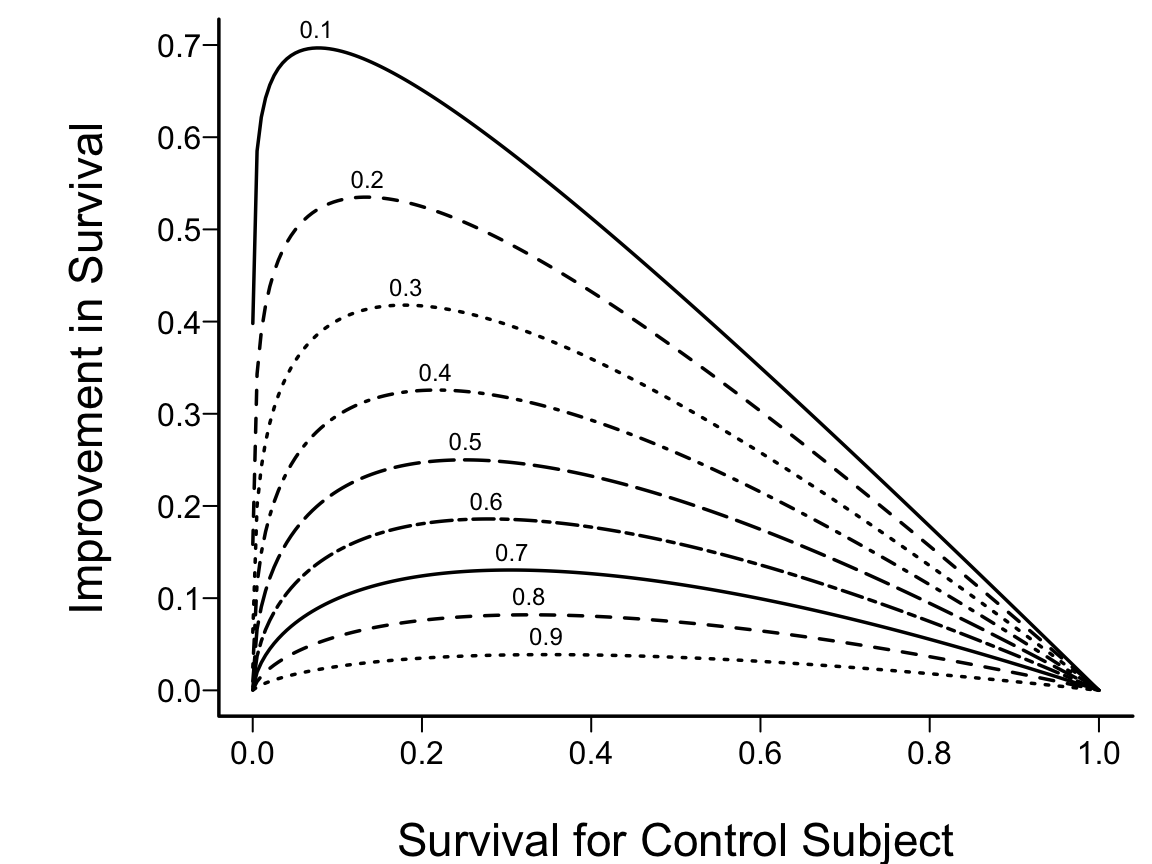Figure 18.1: Absolute clinical benefit as a function of survival in a control subject and the relative benefit (hazard ratio). The hazard ratios are given for each curve.

### 18.2.4 Specific Models

• Exponential:
$\begin{array}{ccc} \lambda(t|X) &=& \lambda \exp(X\beta) \nonumber \\ S(t|X) &=& \exp[-\lambda t \exp(X\beta)] = \exp(-\lambda t)^{\exp(X\beta)} \end{array}$ $\begin{array}{ccc} E\{T|X\} &=& 1/[\lambda \exp(X\beta)] \nonumber \\ T_{0.5}|X &=& (\log 2)/[\lambda \exp(X\beta)] \end{array}$
• Weibull:
$\begin{array}{ccc} \lambda(t|X) &=& \alpha\gamma t^{\gamma-1} \exp(X\beta) \nonumber \\ \Lambda(t|X) &=& \alpha t^{\gamma} \exp(X\beta) \nonumber \nonumber \\ S(t|X) &=& \exp[-\alpha t^{\gamma} \exp(X\beta)] \\ &=& [\exp(-\alpha t^{\gamma})]^{\exp(X\beta)} \nonumber \end{array}$

$T_{0.5}|X=\{\log 2 / [\alpha \exp(X\beta)]\}^{1/\gamma} .$

For numerical reasons, re-write:

$\begin{array}{ccc} S(t|X) &=& \exp(-\Lambda(t|X)) , {\rm\ \ \ where} \nonumber \\ \Lambda(t|X) &=& \exp(\gamma \log t + X\beta) \end{array} \tag{18.1}$

See also spline hazard models Kooperberg et al. (1995) and the generalized gamma distribution .

### 18.2.5 Assessment of Model FitFigure 18.2: PH Model with one binary predictor. $$Y$$-axis is $$\log \lambda(t)$$ or $$\log \Lambda(t)$$. For $$\log \Lambda(t)$$, the curves must be non-decreasing. For $$\log \lambda(t)$$, they may be any shape.

If $$\lambda(t)$$ is Weibull, the two curves will be linear if $$\log t$$ is plotted instead of $$t$$ on the $$x$$-axis.Figure 18.3: PH model with one continuous predictor. $$Y$$-axis is $$\log \lambda(t)$$ or $$\log \Lambda(t)$$. For $$\log \Lambda(t)$$, drawn for $$t_{2}>t_{1}$$. The slope of each line is $$\beta_{1}$$.Figure 18.4: PH model with one continuous predictor. $$Y$$-axis is $$\log \lambda(t)$$ or $$\log \Lambda(t)$$. For $$\log \lambda$$, the functions need not be monotonic.Figure 18.5: Regression assumptions, linear additive PH or AFT model with two predictors. For PH, $$Y$$-axis is $$\log \lambda(t)$$ or $$\log \Lambda(t)$$ for a fixed $$t$$. For AFT, $$Y$$-axis is $$\log(T)$$.
• Weibull: Stratify on $$X$$, plot $$\log \Lambda_{\rm KM}(t|X {\rm\ stratum})$$ vs. $$\log t$$.
• Assesses PH in addition to shape assumptions–all curves should be parallel as well as straight.

## 18.3 Accelerated Failure Time Models

### 18.3.1 Model

• Specifies that predictors act multiplicatively on failure time
• Alters rate subject proceeds along time axis

$S(t|X) = \psi(\frac{\log(t)-X\beta}{\sigma}) \tag{18.2}$

$\begin{array}{ccc} \frac{\log(T)-X\beta}{\sigma} &\sim& \psi \\ \log(T) = X\beta + \sigma\epsilon \\ \epsilon \sim \psi \end{array}$
• Weibull (and exponential) members of PH and AFT

### 18.3.2 Model Assumptions and Interpretation of Parameters

$\psi^{-1}(S(t|X)) = \frac{\log(t)-X\beta}{\sigma} \tag{18.3}$

Letting $$\epsilon \sim \psi$$

$\log(T) = X\beta + \sigma\epsilon$

Check that residuals $$\log(T)-X\hat{\beta} \sim \psi$$ (within scale factor). The assumptions of the AFT model are thus

1. The true form of $$\psi$$ (the distributional family) is correctly specified.
2. In the absence of nonlinear and interaction terms, each $$X_j$$ affects $$\log(T)$$ or $$\psi^{-1}(S(t|X))$$ linearly.
3. Implicit in these assumptions is that $$\sigma$$ is a constant independent of $$X$$.

1-unit change in $$X_{j} = \beta_{j}$$ change in $$\log T$$, or increase $$T$$ by factor of $$\exp(\beta_{j})$$.
Median survival time:

$T_{0.5}|X = \exp(X\beta + \sigma \psi^{-1}(0.5))$

### 18.3.3 Specific Models

• Extreme value: $$\psi(u)=\exp(-\exp(u))$$
• Logistic: $$\psi(u)=[1+\exp(u)]^{-1}$$
• Normal: $$\psi(u)=1 - \Phi(u)$$
• Log-normal:

$S(t|X) = 1 - \Phi(\frac{\log(t)-X\beta}{\sigma}),$

• Log-logistic:

$S(t|X) = [1 + \exp(\frac{\log(t) - X\beta}{\sigma})]^{-1}.$

### 18.3.4 Estimation

Works better if $$\sigma$$ parameterized as $$\exp(\delta)$$.

$\begin{array}{ccc} \hat{S}(t|X) &=& \psi(\frac{\log(t)-X\hat{\beta}}{\hat{\sigma}}) \nonumber \\ \hat{T}_{0.5}|X &=& \exp[X\hat{\beta} + \hat{\sigma} \psi^{-1}(0.5)]. \end{array}$

Normal and logistic: $$\hat{T}_{0.5}|X = \exp(X\hat{\beta})$$

$\psi(\frac{\log(t)-X\hat{\beta}}{\hat{\sigma}}\pm z_{1-\alpha/2}\times s)$

### 18.3.5 Residuals

For an AFT model, standardized residuals are simply

$r = (\log(T)-X\hat{\beta})/\sigma \tag{18.4}$

When $$T$$ is right-censored, $$r$$ is right-censored.

### 18.3.6 Assessment of Model FitFigure 18.6: AFT model with one predictor. $$Y$$-axis is $$\psi^{-1}(S(t|X)) = \frac{\log(t)-X\beta}{\sigma}$$. Drawn for $$d>c$$. The slope of the lines is $$\sigma^{-1}$$.Figure 18.7: AFT model with one continuous predictor. $$Y$$-axis is $$\psi^{-1}(S(t|X)) = \frac{\log(t)-X\beta}{\sigma}$$. Drawn for $$t_{2}>t_{1}$$. The slope of each line is $$\beta_{1}/\sigma$$ and the difference between the lines is $$\frac{1}{\sigma}\log(t_{2}/t_{1})$$.
 Group 1 143 164 188 188 190 192 206 209 213 216 220 227 230 234 246 265 304 216$$^{+}$$ 244$$^{+}$$ Group 2 142 156 163 198 205 232 232 233 233 233 233 239 240 261 280 280 296 296 323 204$$^{+}$$ 344$$^{+}$$
Code
spar(mfrow=c(2,2), top=1, bot=2, mgp=c(2.75, .365, 0))
getHdata(kprats)
kprats$group <- factor(kprats$group, 0:1, c('Group 1', 'Group 2'))
S <- with(kprats, Surv(t, death))
f <- npsurv(S ~ group, type="fleming", data=kprats)
survplot(f, n.risk=TRUE, conf='none',
label.curves=list(keys='lines'), levels.only=TRUE)
title(sub="Nonparametric estimates", adj=0, cex=.7)
# Check fits of Weibull, log-logistic, log-normal
xl <- c(4.8, 5.9)
survplot(f, loglog=TRUE, logt=TRUE, conf="none", xlim=xl,
label.curves=list(keys='lines'), levels.only=TRUE)
title(sub="Weibull (extreme value)", adj=0, cex=.7)
survplot(f, fun=function(y)log(y/(1-y)), ylab="logit S(t)",
logt=TRUE, conf="none", xlim=xl,
label.curves=list(keys='lines'), levels.only=TRUE)
survplot(f, fun=qnorm, ylab="Inverse Normal S(t)",
logt=TRUE, conf="none",
xlim=xl,cex.label=.7,
label.curves=list(keys='lines'), levels.only=TRUE)
title(sub="Log-normal", adj=0, cex=.7)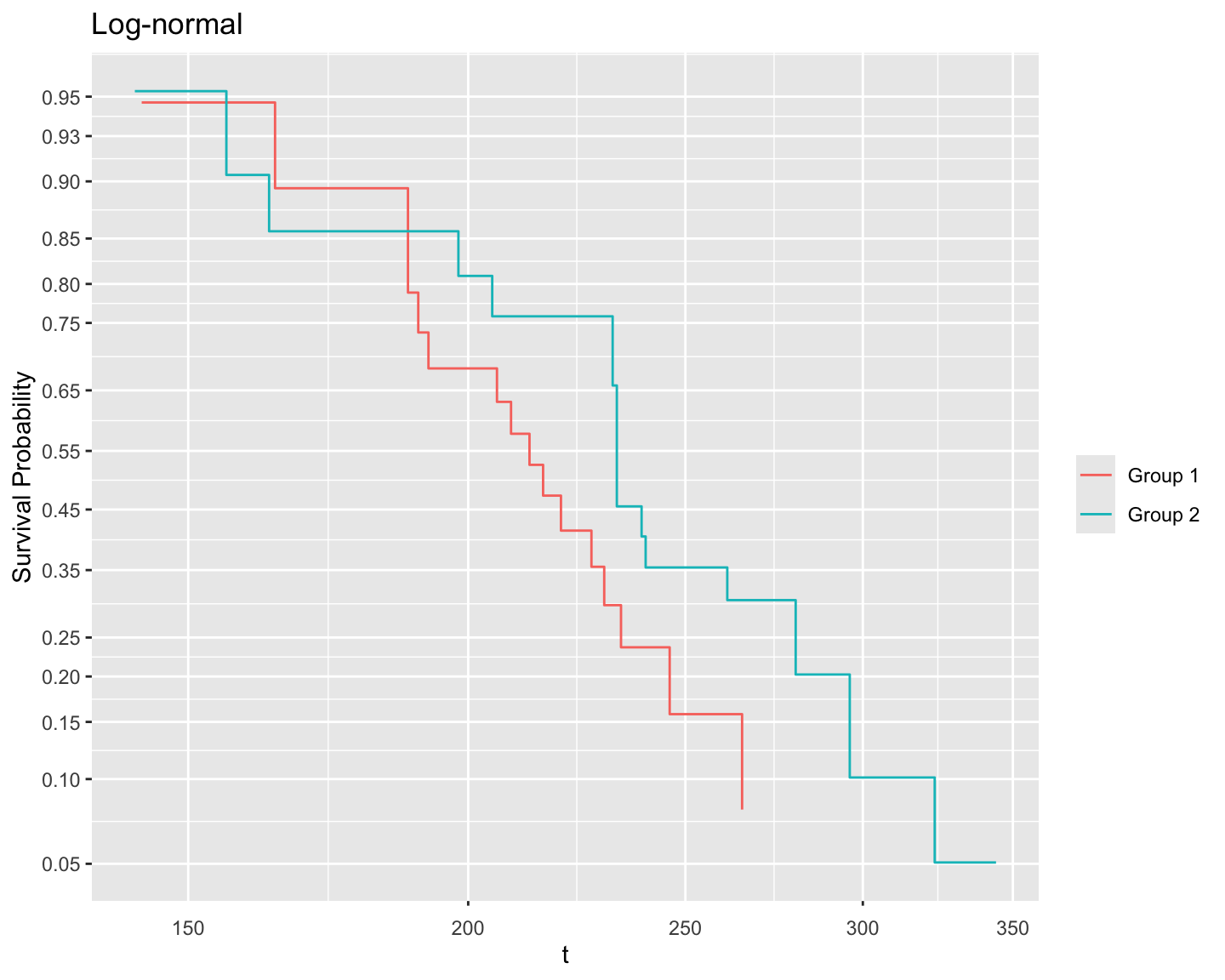Figure 18.8: Altschuler-Nelson-Fleming-Harrington nonparametric survival estimates for rats treated with DMBA (Pike, 1966), along with various transformations of the estimates for checking distributional assumptions of 3 parametric survival models.

Fit Weibull (in aft form), log-logistic, and log-normal models.

Code
fw <- psm(S ~ group, data=kprats, dist='weibull')
fl <- psm(S ~ group, data=kprats, dist='loglogistic',
y=TRUE)
fn <- psm(S ~ group, data=kprats, dist='lognormal')
cat('**Weibull default form**:\n\n')

Weibull default form:

Code
latex(fw)
$\Pr(T\geq t) = \exp[-\exp( \frac{\log(t)-X\beta}{0.1832976} )]~\text{where}$ $\begin{array} \lefteqn{X\hat{\beta}=}\\ & & 5.450859 \\ & & +0.131983[\text{Group\ 2}] \\ \end{array}$

$[c]=1~\text{if subject is in group}~c,~0~\text{otherwise}$

Code
cat('**Weibull PH form**:\n\n')

Weibull PH form:

Code
latex(pphsm(fw))
$\Prob(T\geq t) = \exp(-t^{ 5.455608 } \exp(X\hat{\beta}))~\text{where}~~$ $\begin{array} \lefteqn{X\hat{\beta}=}\\ & & -29.73775 \\ & & -0.7200475[\text{Group\ 2}] \\ \end{array}$

$[c]=1~\text{if subject is in group}~c,~0~\text{otherwise}$

Code
cat('**Log-logistic**:\n\n')

Log-logistic:

Code
latex(fl)
$\Pr(T\geq t) = [1+\exp( \frac{\log(t)-X\beta}{0.1159753} )]^{-1}~\text{where}$ $\begin{array} \lefteqn{X\hat{\beta}=}\\ & & 5.375675 \\ & & +0.1051005[\text{Group\ 2}] \\ \end{array}$

$[c]=1~\text{if subject is in group}~c,~0~\text{otherwise}$

Code
cat('**Log-normal**')

Log-normal

Code
latex(fn)
$\Pr(T\geq t) = 1-\Phi( \frac{\log(t)-X\beta}{0.2100184} )~\text{where}$ $\begin{array} \lefteqn{X\hat{\beta}=}\\ & & 5.375328 \\ & & +0.0930606[\text{Group\ 2}] \\ \end{array}$

$[c]=1~\text{if subject is in group}~c,~0~\text{otherwise}$Group effects from three survival models
• More general approach to verifying distributional assumptions:
• Plot nonparametric estimate of survival distribution of $$r$$
• Superimpose theoretical standardized distribution
• Can get distribution of residuals separately by strata — should all have same standardized distribution (e.g., same $$\sigma$$)
Code
survplot(f, conf.int=FALSE,
levels.only=TRUE, label.curves=list(keys='lines'))
survplot(fl, add=TRUE, label.curves=FALSE, conf.int=FALSE)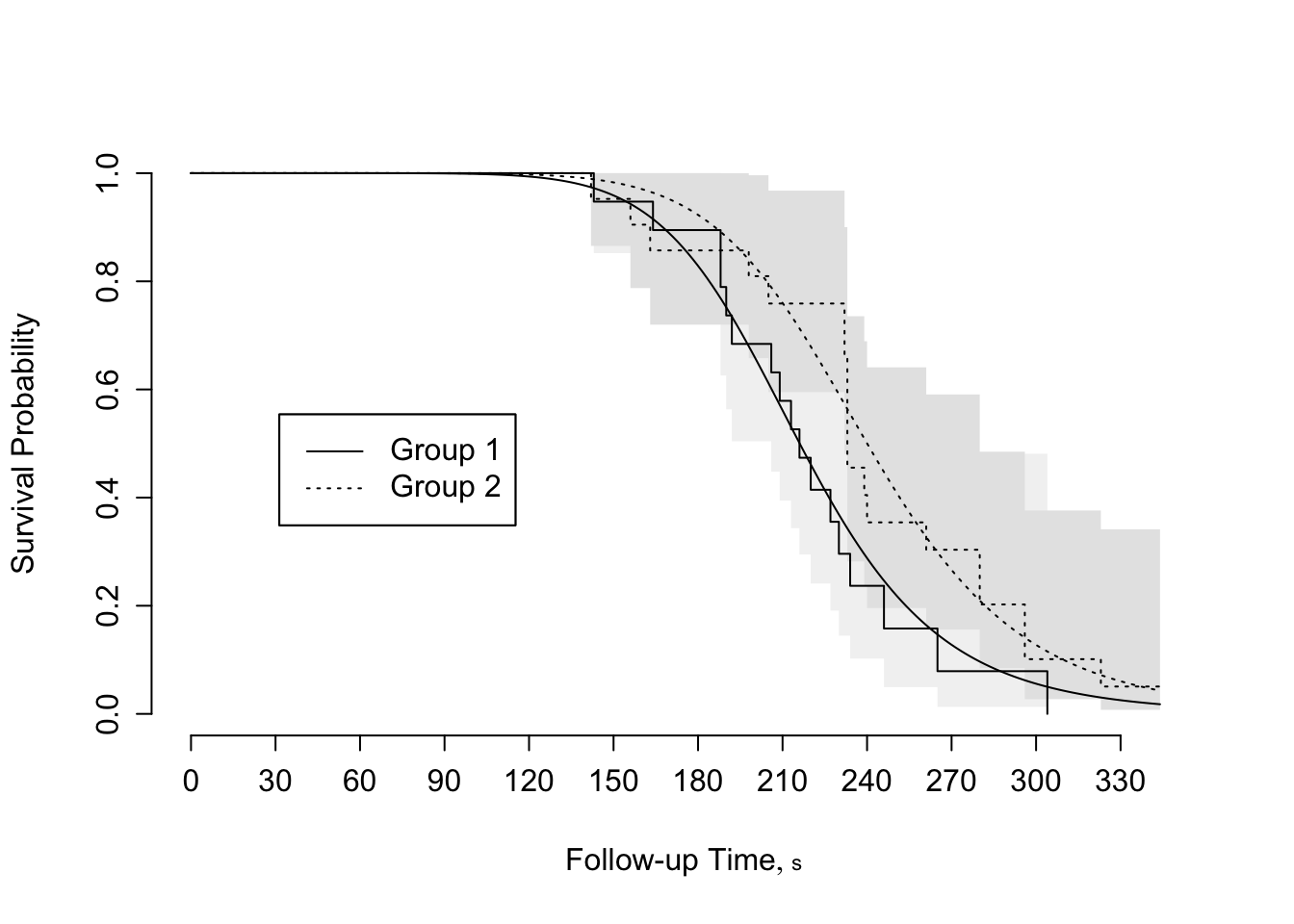Figure 18.9: Agreement between fitted log-logistic model and nonparametric survival estimates for rat vaginal cancer data
Code
r <- resid(fl, 'cens')
survplot(npsurv(r ~ group, data=kprats),
conf='none', xlab='Residual',
label.curves=list(keys='lines'), levels.only=TRUE)
survplot(npsurv(r ~ 1), conf='none', add=TRUE, col='red')
lines(r, lwd=1, col='blue')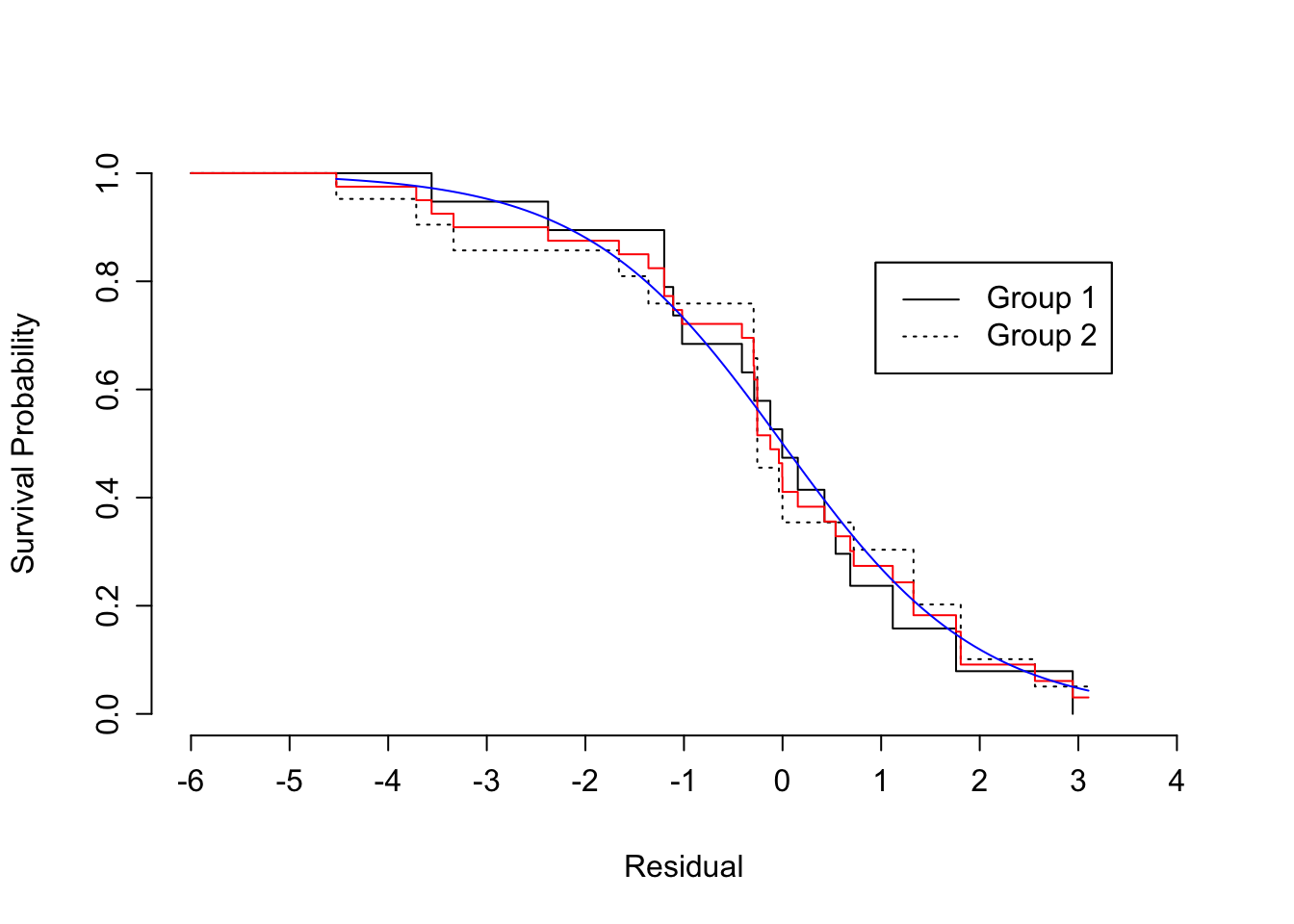Figure 18.10: Kaplan-Meier estimates of distribution of standardized, censored residuals from the log-logistic model, along with the assumed standard log-logistic distribution (blue). Red step function is the estimated distribution of all residuals; black step functions are the estimated distributions of residuals stratified by group, as indicated.

Derive R code for median, mean, hazard, survival functions

Code
med   <- Quantile(fl)
med
function (q = 0.5, lp = NULL, parms = -2.15437773933124)
{
names(parms) <- NULL
f <- function(lp, q, parms) lp + exp(parms) * logb(q/(1 -
q))
names(q) <- format(q)
drop(exp(outer(lp, q, FUN = f, parms = parms)))
}
<environment: namespace:rms>
Code
meant <- Mean(fl)
meant
function (lp = NULL, parms = -2.15437773933124)
{
names(parms) <- NULL
if (exp(parms) > 1)
rep(Inf, length(lp))
else exp(lp) * pi * exp(parms)/sin(pi * exp(parms))
}
<environment: namespace:rms>
Code
haz   <- Hazard(fl)
haz
function (times = NA, lp = NULL, parms = -2.15437773933124)
{
t.trans <- logb(times)
t.deriv <- 1/times
scale <- exp(parms)
names(t.trans) <- format(times)
t.deriv/scale/(1 + exp(-(t.trans - lp)/scale))
}
<environment: namespace:rms>
Code
surv  <- Survival(fl)
surv
function (times = NULL, lp = NULL, parms = -2.15437773933124)
{
1/(1 + exp((logb(times) - lp)/exp(parms)))
}
<environment: namespace:rms>

Show fitted hazard function from log-logistic, and add median survival time to graph

Code
spar(ps=9,top=1,bot=1,left=1,mgp=c(2.75,.365,0))
# Plot estimated hazard functions and add median
# survival times to graph
survplot(fl, group, what="hazard")
# Compute median survival time
m <- med(lp=predict(fl,
data.frame(group=levels(kprats$group)))) m  1 2 216.0857 240.0328  Code med(lp=range(fl$linear.predictors))
 216.0857 240.0328
Code
m <- format(m, digits=3)
text(68, .02, paste("Group 1 median: ", m,"\n",
"Group 2 median: ", m, sep=""))
# Compute survival probability at 210 days
xbeta <- predict(fl,
data.frame(group=c("Group 1","Group 2")))
surv(210, xbeta)
        1         2
0.5612718 0.7599776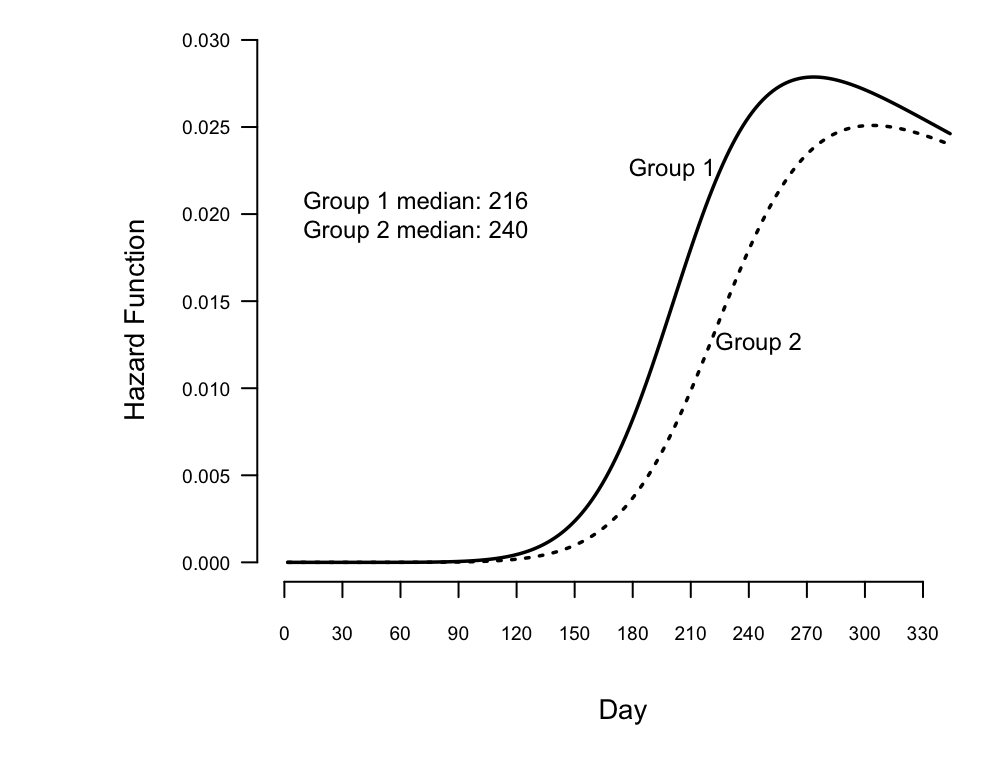Figure 18.11: Estimated hazard functions for log-logistic fit to rat vaginal cancer data, along with median survival times

### 18.3.7 Validating the Fitted Model

• Check distributional shape
• Group predicted $$t$$-year survival and plot Kaplan-Meier estimate at $$t$$ vs. mean predicted $$\hat{S}$$
• Cox-Snell residuals — check against $$U[0,1]$$
• loess smooth of $$F(T | X) - 0.5 F(C | X)$$ against $$X \hat{\beta}$$ or $$\frac{2 F(T | X)}{F(C | X)}$$ vs. $$X \hat{\beta}$$ if $$C$$ is known

See the val.surv function in the rms package.